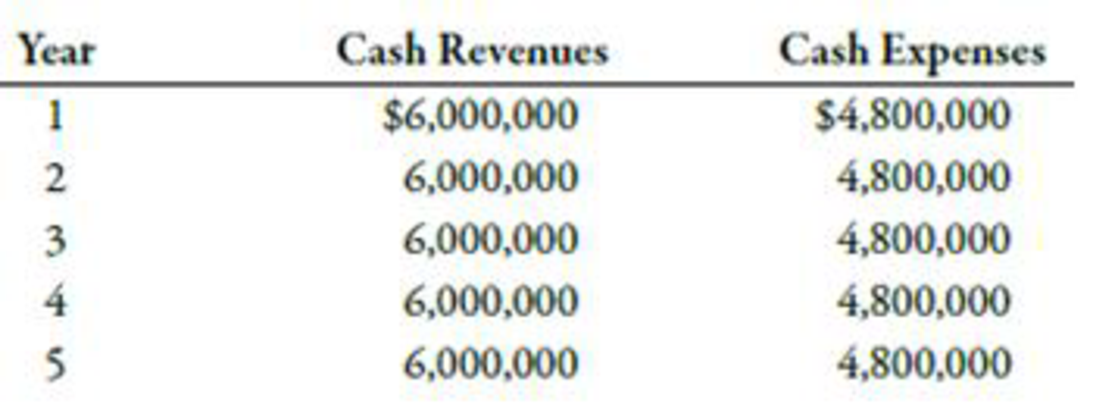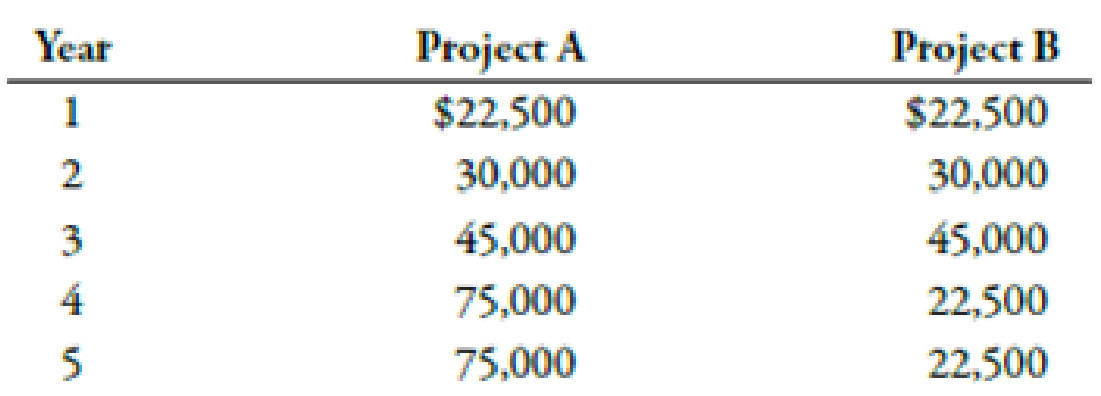Chapter 12, Problem 32E### Managerial Accounting: The Corners...

7th Edition
Maryanne M. Mowen + 2 others
ISBN: 9781337115773

#### Solutions

Chapter
Section### Managerial Accounting: The Corners...

7th Edition
Maryanne M. Mowen + 2 others
ISBN: 9781337115773
Textbook Problem
183 views

# Accounting Rate of ReturnEach of the following scenarios is independent. Assume that all cash flows are after-tax cash flows.Cobre Company is considering the purchase of new equipment that will speed up the process for extracting copper. The equipment will cost $3,600,000 and have a life of 5 years with no expected salvage value. The expected cash flows associated with the project are as follows:Emily Hansen is considering investing in one of the following two projects. Either project will require an investment of$75,000. The expected cash revenues minus cash expenses for the two projects follow. Assume each project is depreciable.Suppose that a project has an ARR of 30% (based on initial investment) and that the average net income of the project is $120,000.Suppose that a project has an ARR of 50% and that the investment is$150,000.Required:Compute the ARR on the new equipment that Cobre Company is considering.CONCEPTUAL CONNECTION Which project should Emily Hansen choose based on the ARR? Notice that the payback period is the same for both investments (thus equally preferred). Unlike the payback period, explain why ARR correctly signals that one project should be preferred over the other.How much did the company in Scenario c invest in the project?What is the average net income earned by the project in Scenario d?

1.

To determine

Find out the ARR for the new equipment of Company C.

Explanation

Accounting Rate of Return:

A method that measures returns from an investment in terms of income instead of cash flow is known as accounting rate of return. It is a non-discounting model of capital investment decision.

Use the following formula to calculate accounting rate of return (ARR):

ARR=AveragenetincomeInitialinvestment

Substitute $480,0001 for average net income and$3,600,000 for initial investment in the above formula.

ARR=$480,000$3,600,000=13.3%

Therefore, accounting rate of return is 13.13%.

Working Note:

1. Calculation of depreciation:

Depreciation=CostSalvagev

2.

To determine

Identify the project to be chosen on the basis of ARR. Also explain the accuracy of ARR in the selection one project over the other.

3.

To determine

Calculate the amount to be invested in the project.

4.

To determine

Calculate average net income earned.

### Still sussing out bartleby?

Check out a sample textbook solution.

See a sample solution

#### The Solution to Your Study Problems

Bartleby provides explanations to thousands of textbook problems written by our experts, many with advanced degrees!

Get Started

#### Find more solutions based on key concepts